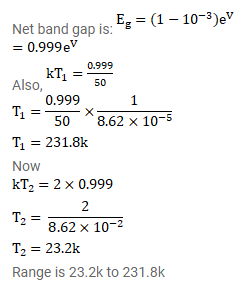# A semiconductor material has a band gap of 1eV.

Question:

A semiconductor material has a band gap of 1eV. Acceptor impurities are doped into it which create acceptor levels 1 meV above the valence band. Assume that the transition from one energy level to the other is almost forbidden if $k T$ is less than $1 / 50$ of the energy gap. Also, if $k T$ is more than twice the gap. The upper levels have maximum population. The temperature of the semiconductor is increased from oK. The concentration of the holes increases with temperature and after a certain temperature it becomes approximately constant. As the temperature is further increased, the hole concentration again starts increasing at a certain temperature. Find the order of the temperature range in which the hole concentration remains approximately constant.

Solution: